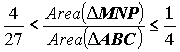### Problem 1

Given an acute triangle ABC, let D be the midpoint of the arc BC of the circumcircle around the triangle ABC, not containing the point A. The points which are symmetric to D with respect to the line BC and the circumcentre O are denoted by E and F, respectively. Finally, let K be the midpoint of the segment [EA]. Prove that:
a) The circle passing through the midpoints of the sides of the triangle ABC, also passes through K;
b) the line passing through K and the midpoint of the segment [BC] is perpendicular to the line AF.

### Problem 2

Let p > 2 be a prime number, such that 3 divides p - 2. Let:

S = { y2 - x3 - 1 | x and y are integers, 0 =< x, y =< p - 1}.

Prove that at most p-1 elements of the set S are divisible by p.

### Problem 3

Let ABC be an acute triangle, and let M, N and P be the feet of the perpendiculars drawn from the centriod G of the triangle ABC towards its sides AB, BC and CA, respectively. Prove that:### Problem 4

Let 0=< x1=< x2 =< x3=< ... =< xn=< ... be a non-decreasing sequence of non-negative integers, such that for every k, k >= 0, the number of terms of the sequence which are less than or equal to k is finite, and let that number be denoted by yk. Prove that for all positive integers m and n it holds: